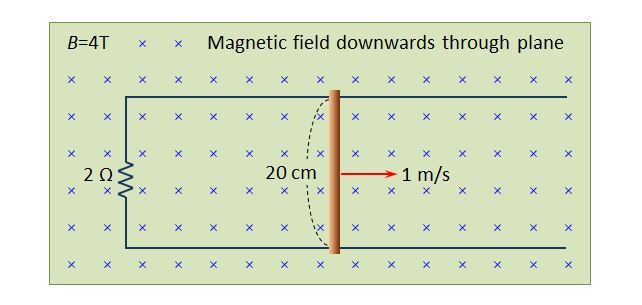# Induced electromotive forceThe above diagram is a uniform magnetic field with intensity $4\text{ T}.$ The direction of the magnetic field is downwards through the plane on which a conducting wire and a $20\text{ cm}$ metal stick are placed as shown above. If we drag the metal stick on the conducting wire to the right at a constant speed of $1 \text{ m/s},$ then what is the current intensity flowing in the resistor with a resistance of $2 \text{ }\Omega?$

×